# crplot

## Introduction

The crplot function is part of the conf package. It generates a two-dimensional confidence region plot for the specified two-parameter parametric distribution, fitted to a dataset.

## Installation Instructions

The crplot function is accessible following installation of the conf package:

install.packages("conf")
library(conf)

## Example

The dataset for ball bearing failure times, given by Lieblein and Zelen1, is used throughout this example. Its fit to the Weibull distribution, including the confidence region illustrated next, is also explained in depth in the Reliability textbook by Leemis2.

After reading ball bearing failure times (in millions of revolutions) into the vector ballbearing, crplot is called using arguments for the Weibull distribution, and a level of significance $$\alpha = 0.05$$ to yield a $$95\%$$ confidence region.

library(conf)
ballbearing <- c(17.88, 28.92, 33.00, 41.52, 42.12, 45.60, 48.48, 51.84,
51.96, 54.12, 55.56, 67.80, 68.64, 68.64, 68.88, 84.12,
93.12, 98.64, 105.12, 105.84, 127.92, 128.04, 173.40)
crplot(dataset = ballbearing, alpha = 0.05, distn = "weibull")
#>  "Confidence region plot complete; made using 102 boundary points."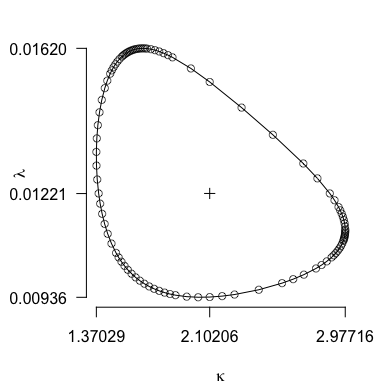In addition to the $$95\%$$ confidence region plot, output informs the user that its smoothing boundary search heuristic (default heuristic) uses 102 points to complete the plot. The maximum likelihood estimator values are plotted as a + within the confidence region.

Valuable perspective is often given if the origin is included within a plot. The argument origin = TRUE in the plot below invokes this change. Alternatively, the user can specify a unique frame of reference using optional xlim and/or ylim arguments. Two additional modifications complete the plot below: its points are hidden using pts = FALSE, and significant figures for the respective horizontal and vertical axes are specified using sf = c(2, 4).

crplot(dataset = ballbearing, alpha = 0.05, distn = "weibull",
pts = FALSE, sf = c(2, 4), origin = TRUE)
#>  "Confidence region plot complete; made using 102 boundary points."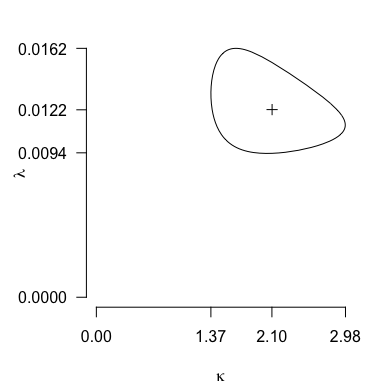Setting info = TRUE allows the user to access data pertinent to the resulting plot for subsequent analysis and/or plot customization. This is shown next; also note the assignment x <- crplot(...), which is necessary to collect plot data for future use.

x <- crplot(dataset = ballbearing, alpha = 0.05, distn = "weibull",
pts = FALSE, sf = c(2, 4), origin = TRUE, info = TRUE)
#>  "Confidence region plot complete; made using 102 boundary points."
names(x)
#>  "kappa"     "lambda"    "phi"       "kappahat"  "lambdahat"

Displaying names(x) confirms that info = TRUE directs crplot to return a list with respective arguments "kappa", "lambda", and "phi". The "kappa" and "lambda" parameters correspond to the plot horizontal and vertical axes values. They are the Weibull distribution shape and scale parameters, respectively. These parameters update appropriately when fitting the data to the inverse Gaussian distribution (returning mean and shape parameters, "mu" and "lambda", respectively). The third list argument, "phi", gives angles in radians relative to the MLE, corresponding to each plot point. These "phi" ($$\phi$$) values determined plot points using Jaeger’s radial profile log likelihood technique3.

Custom plots and analysis are possible using the data returned when info = TRUE. Two examples follow. The first shades the confidence region and alters its border line-type.

# with confidence region data stored in x, it is now available for custom graphics
plot(x$kappa, x$lambda, type = 'l', lty = 5, xlim = c(0, 3), ylim = c(0, 0.0163),
xlab = expression(kappa), ylab = expression(lambda))
polygon(x$kappa, x$lambda, col = "gray80", border = NA)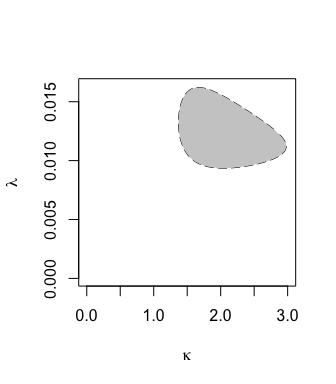The second example will analyze $$\phi$$ angles (with respect to the MLE) used to create the confidence region plot. This is done with two plots.

The first of the two plots illustrates the confidence region plot, with segments radiating from its MLE at applicable $$\phi$$ values. Note the greater density of $$\phi$$ angles for plot regions with greater curvature. This is a consequence of the smoothing search heuristic (heuristic = 1) plotting algorithm used as the default plotting method for crplot.

The second plot is a perspective of phi values numerically using a cumulative distribution function. Note how most $$\phi$$ angles are near horizontal orientation ($$0$$ and $$\pi$$ radians, respectfully). This is a consequence of the vastly different scales for its respective parameters, $$\kappa$$ and $$\lambda$$. The actual $$\phi$$ angles required to assemble the confidence region plot can vary greatly from their apparent angles due to scale differences between axes.

# record MLE values (previously output to screen when info = TRUE) & reproduce CR plot
kappa.hat <- 2.10206
lambda.hat <- 0.01221
crplot(dataset = ballbearing, alpha = 0.05, distn = "weibull", xlab = "shape",
ylab = " ", main = paste0("Confidence Region"),
pts = FALSE, mlelab = FALSE, sf = c(2, 4), origin = TRUE)
#>  "Confidence region plot complete; made using 102 boundary points."
par(xpd = TRUE)
text(-1.2, 0.007, "scale")

# 1st analysis plot: overlay phi angles (as line segments) on the confidence region plot
par(xpd = FALSE)
segments(rep(kappa.hat, length(x$phi)), rep(lambda.hat, length(x$phi)),
x1 = kappa.hat * cos(x$phi) + kappa.hat, y1 = kappa.hat * sin(x$phi) + lambda.hat, lwd = 0.2)

# 2nd analysis plot: CDF of phi angles reveals most are very near 0 (2pi) and pi
plot.ecdf(x$phi, pch = 20, cex = 0.1, axes = FALSE, xlab = expression(phi), ylab = "", main = "CDF") axis(side = 1, at = round(c(0, pi, 2*pi), 2)) axis(side = 2, at = c(0, 1), las = 2)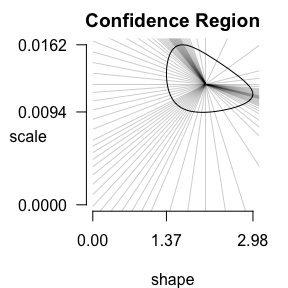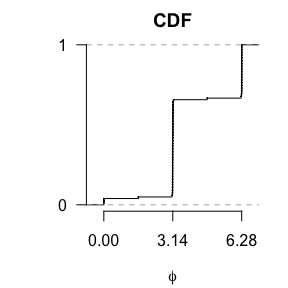All confidence region plots to this point are made using the default smoothing search heuristic (heuristic = 1). It plots more points in border regions with higher curvature, and does so until a maximum apparent angle constraint between three successive points is met (apparent angles assume a square plot area and account for axes scale differences). That constraint (default $$5^\circ$$) is customizable using the optional maxdeg argument to yield plots using more points for greater definition (maxdeg values < 5) or with less points at a reduced resolution (maxdeg values > 5). Lower resolution graphics sacrifice detail and are less computationally expensive. This is significant if generating a large sample of confidence regions (i.e. hundreds of confidence regions supporting bootstrap analysis repetitions, or a large pairwise matrix evaluation). The default maxdeg = 5 is appropriate for most circumstances. Values of maxdeg < $$3^\circ$$ are not permitted to avoid numeric approximation complications implicit with populating too many confidence region boundary points. The plots below illustrate confidence region plot results with increased and decreased resolution. crplot(dataset = ballbearing, alpha = 0.05, distn = "weibull", maxdeg = 3, main = "maxdeg = 3") #>  "Confidence region plot complete; made using 164 boundary points." crplot(dataset = ballbearing, alpha = 0.05, distn = "weibull", maxdeg = 3, main = "maxdeg = 3 (pts hidden)", pts = FALSE) #>  "Confidence region plot complete; made using 164 boundary points." crplot(dataset = ballbearing, alpha = 0.05, distn = "weibull", maxdeg = 20, main = "maxdeg = 20") #>  "Confidence region plot complete; made using 32 boundary points." crplot(dataset = ballbearing, alpha = 0.05, distn = "weibull", maxdeg = 20, main = "maxdeg = 20 (pts hidden)", pts = FALSE) #>  "Confidence region plot complete; made using 32 boundary points."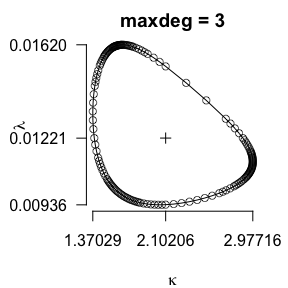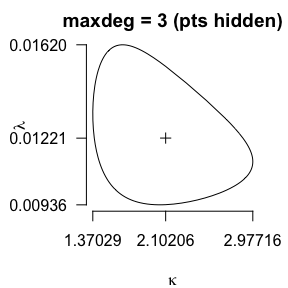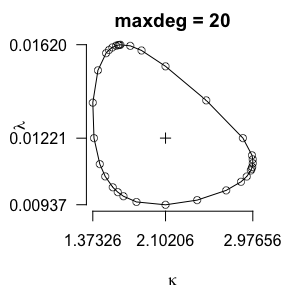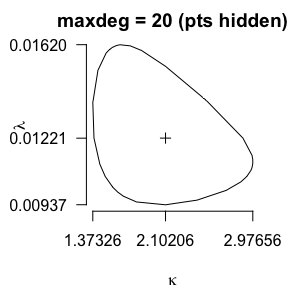An alternative plot heuristic is available using the optional argument heuristic = 0. It plots a predetermined number of confidence region points (user specified using ellipse_n) in a roughly uniform fashion along its boundary using an elliptic-oriented plotting technique. Its name references the method its algorithm uses to draw an ellipse—known as the parallelogram method; a result of the Theorem of Steiner4—that identifies approximately equidistant points along the confidence region boundary regardless of the relative scales of its axes. The next pair of plots illustrate differences between the default smoothing search plot heuristic and the elliptic-oriented alternative heuristic (heuristic = 0). They also illustrate a fit to the inverse Gaussian distribution, rather than the Weibull distribution shown previously. crplot(dataset = ballbearing, alpha = 0.05, distn = "invgauss", sf = c(2, 2), main = "default; heuristic = 1") #>  "Confidence region plot complete; made using 103 boundary points." crplot(dataset = ballbearing, alpha = 0.05, distn = "invgauss", sf = c(2, 2), heuristic = 0, ellipse_n = 100, main = "heuristic = 0") #>  "Confidence region plot complete; made using 100 boundary points."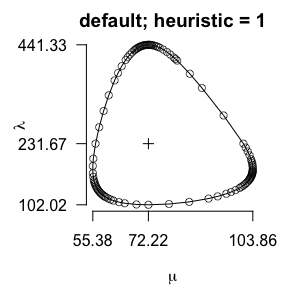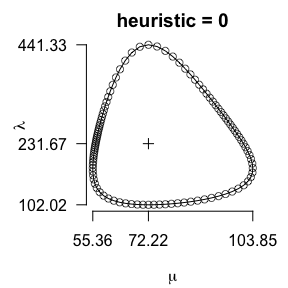An ellipse_n value must always accompany use of heuristic = 0. Additionally, ellipse_n must be a positive integer multiple of four $$\geq 8$$. This requirement enables its algorithm to exploit computational efficiencies associated with ellipse symmetry throughout its respective quadrants. Plot heuristics are combined if an ellipse_n value $$\geq 8$$ is given under default plot conditions, or equivalently heuristic = 1. In this case, crplot implements the heuristics in sequence. It begins plotting the confidence region using ellipse_n points through the heuristic = 0 plot algorithm, and then augments points as necessary to meet maxdeg constraints corresponding to its default smoothing search heuristic. Although its steps are hidden from view when using this approach, they are shown below for clarity. The final plot (combination: step 2) augments the step 1 plot points (showing heuristic = 0, ellipse_n = 16 results) according to the smoothing search heuristic with a maximum angle tolerance of maxdeg = 10. crplot(dataset = ballbearing, alpha = 0.05, distn = "invgauss", sf = c(2, 2), heuristic = 0, ellipse_n = 40, main = "combination: step 1") #>  "Confidence region plot complete; made using 40 boundary points." crplot(dataset = ballbearing, alpha = 0.05, distn = "invgauss", sf = c(2, 2), maxdeg = 10, ellipse_n = 40, main = "combination: step 2") #>  "Confidence region plot complete; made using 68 boundary points."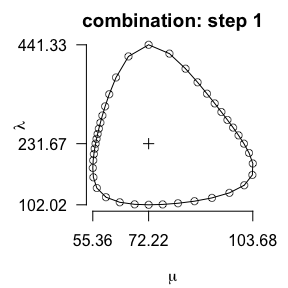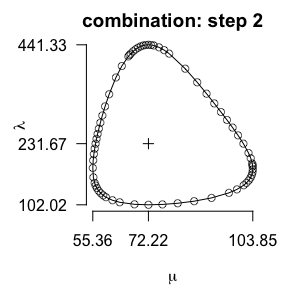The next plot illustrates the default smoothing search heuristic with maxdeg = 10 (matching the above parameterization) for means of comparison with the combined approach above. crplot(dataset = ballbearing, alpha = 0.05, distn = "invgauss", sf = c(2, 2), maxdeg = 10, main = "default (heuristic = 1)") #>  "Confidence region plot complete; made using 52 boundary points."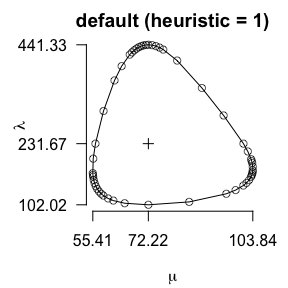## Repairing Radially Inaccessible Regions The default plot technique, known as the smoothing search heuristic and given by heuristic = 1, can fail to achieve its maximum apparent angle constraint (maxdeg, whose default is $$5^\circ$$). This issue arises when plotting non-convex confidence regions whose shape results in multiple confidence region boundary points at select angles from its MLE. This is often a consequence of small sample size and/or small significance level alpha. The optional argument repair corrects such circumstance. Its default is repair = TRUE, however, it is set to repair = FALSE below to illustrate these issues. X <- seq(1, 2.5, by = 0.25) crplot(dataset = X, alpha = 0.01, distn = "gamma", sf = c(2, 2), pts = FALSE, repair = FALSE, main = "without repair") #> Warning in crsmooth(maxdeg = maxdeg, samp = mydata, cen = cen, alpha = #> alpha, : max iteration tolerance hit; working confidence region plot is #> shown, however, maxdeg constraint is not met #>  "Confidence region plot complete; made using 191 boundary points." x <- crplot(seq(1, 2.5, by = 0.25), 0.01, "gamma", sf = c(2, 2), info = TRUE, repair = FALSE, main = "without repair") #> Warning in crsmooth(maxdeg = maxdeg, samp = mydata, cen = cen, alpha = #> alpha, : max iteration tolerance hit; working confidence region plot is #> shown, however, maxdeg constraint is not met #>  "Confidence region plot complete; made using 191 boundary points." index1 <- which(x$kappa == max(x$kappa)) index2 <- which(x$theta == max(x$theta)) lines(c(x$thetahat, x$theta[index1]), c(x$kappahat, x$kappa[index1]), col = "red") lines(c(x$thetahat, x$theta[index2]), c(x$kappahat, x$kappa[index2]), col = "red")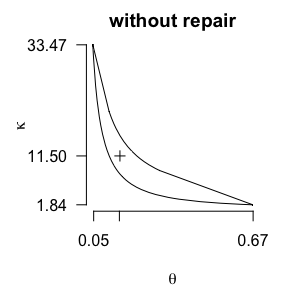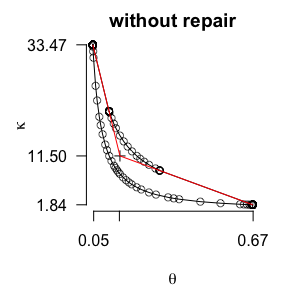The solid red lines in the right figure above represent the boundary to an inaccessible confidence region area. Multiple indicators lead to this conclusion. They include: (1) noticeable and relatively “sharp” vertex angles in the left figure above, (2) the absence of plot points in two “gap” regions along the red line in the right figure above, and (3) the Warning message output specifying the maxdeg constraint is not met. When its default repair = TRUE is kept, the smoothing search heuristic iterates to address each region requiring repair. These successive iterations effectively re-locate the point-of-reference for radial angles away from the MLE and toward the inaccessible region(s) such that uncharted areas become radially accessible. A message notifying use of these “alternate-centerpoint(s)” displays to the screen when this feature of the plot algorithm is employed (shown in the example that follows). Revisiting our previous example, the complete confidence region is successfully plot below given the default argument repair = TRUE is unaltered. The red lines in the example above are also included below for reference. crplot(seq(1, 2.5, by = 0.25), 0.01, "gamma", sf = c(2, 2), pts = FALSE, main = "with repair") #> alternate-centerpoint(s) used to repair plot regions inaccessible via a radial angle from its MLE #>  "Confidence region plot complete; made using 348 boundary points." crplot(seq(1, 2.5, by = 0.25), 0.01, "gamma", sf = c(2, 2), main = "with repair") #> alternate-centerpoint(s) used to repair plot regions inaccessible via a radial angle from its MLE #>  "Confidence region plot complete; made using 348 boundary points." lines(c(x$thetahat, x$theta[index1]), c(x$kappahat, x$kappa[index1]), col = "red") lines(c(x$thetahat, x$theta[index2]), c(x$kappahat, x\$kappa[index2]), col = "red")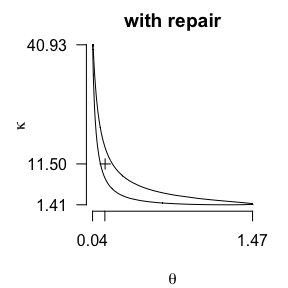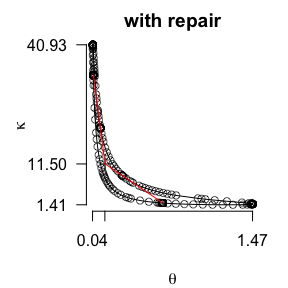Two notable difference between the above result and the previous attempt when repair = FALSE are: (1) the number of plot points has increased from 191 to 348, and (2) the axes ranges have increased substantially. Both of these impacts are the result of additional “repair” points augmenting the previous result.

The absence of the Warning message seen when maxdeg constraints are not met is indicative that they are met; the maximum apparent angle between any two successive plot points is within the maxdeg apparent angle tolerance of $$5 ^\circ$$. This is true even at the bottom-right and top-left confidence region extremes where it appears a sharp point is possible. This fact is confirmed by zooming-in on those respective areas below:

crplot(seq(1, 2.5, by = 0.25), 0.01, "gamma", sf = c(2, 2), pts = TRUE, main = "max(theta) zoom", xlim = c(1.25, 1.5), ylim = c(1.4, 2.4))
#> alternate-centerpoint(s) used to repair plot regions inaccessible via a radial angle from its MLE
#>  "Confidence region plot complete; made using 348 boundary points."
crplot(seq(1, 2.5, by = 0.25), 0.01, "gamma", sf = c(2, 2), pts = TRUE, main = "max(kappa) zoom", xlim = c(0.04, 0.05), ylim = c(37, 41))
#> alternate-centerpoint(s) used to repair plot regions inaccessible via a radial angle from its MLE
#>  "Confidence region plot complete; made using 348 boundary points."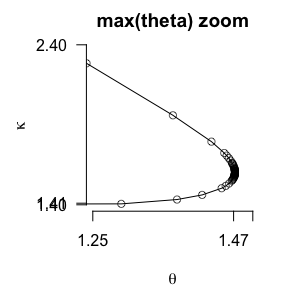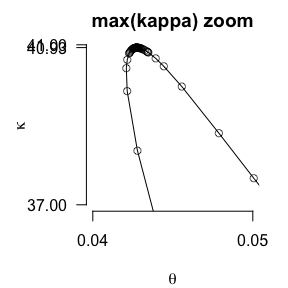The final examples below demonstrate how repairs are necessary for some (log logistic and Weibull distributions) but not all (normal) distributions given this particular small sample of $$n = 2$$ values.

  x <- crplot(c(2, 2.5), 0.01, "llogis", sf = c(2, 2), info = TRUE, pts = FALSE, main = "llogis")
#> alternate-centerpoint(s) used to repair plot regions inaccessible via a radial angle from its MLE
#> Warning in crplot(c(2, 2.5), 0.01, "llogis", sf = c(2, 2), info = TRUE, :
#> small sample size is ill-suited to invoke the asymptotic properties assumed
#> by the confidence region plot
#>  "Confidence region plot complete; made using 311 boundary points."
x <- crplot(c(2, 2.5), 0.01, "weibull", sf = c(2, 2), info = TRUE, pts = FALSE, main = "weibull")
#> alternate-centerpoint(s) used to repair plot regions inaccessible via a radial angle from its MLE
#> Warning in crplot(c(2, 2.5), 0.01, "weibull", sf = c(2, 2), info = TRUE, :
#> small sample size is ill-suited to invoke the asymptotic properties assumed
#> by the confidence region plot
#>  "Confidence region plot complete; made using 313 boundary points."
x <- crplot(c(2, 2.5), 0.01, "norm", sf = c(2, 2), info = TRUE, pts = FALSE, main = "weibull")
#> Warning in crplot(c(2, 2.5), 0.01, "norm", sf = c(2, 2), info = TRUE,
#> pts = FALSE, : small sample size is ill-suited to invoke the asymptotic
#> properties assumed by the confidence region plot
#>  "Confidence region plot complete; made using 100 boundary points."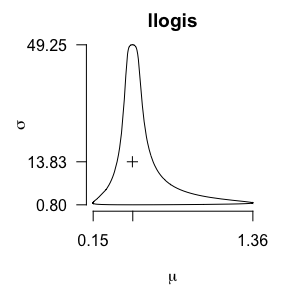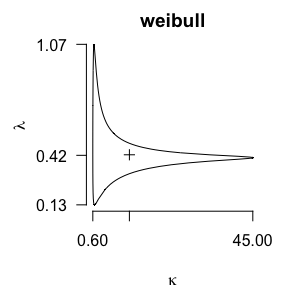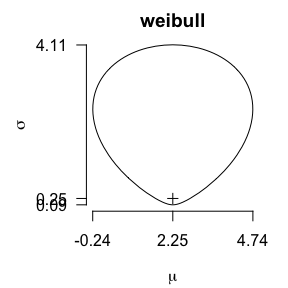Overriding the default with repair = FALSE is not typically recommended, but possible for two reasons. First, repairs require additional computation time that circumstance may warrant avoiding for a quicker (albeit less-precise) solution. Second, occasionally crplot fails to complete a confidence region plot due an R uniroot or other numeric failure. In such cases, turning off repairs can enable an otherwise unobtainable plot to return to the user.

1. Lieblein, J., and Zelen, M. (1956), Statistical Investigation of the Fatigue Life of Deep-Groove Ball Bearings, Journal of Research of the National Bureau of Standards, 57, 273–316

2. Leemis, L. (1995), Reliability: Probabilistic Models and Statistical Methods Second Edition, Prentice-Hall Inc., 345–251

3. Jaeger, A. (2016), “Computation of Two- and Three-Dimensional Confidence Regions With the Likelihood Ratio”, The American Statistician, 70, 395–398

4. Meserve, B. E. (2014), Fundamental Concepts of Geometry, Courier Corporation